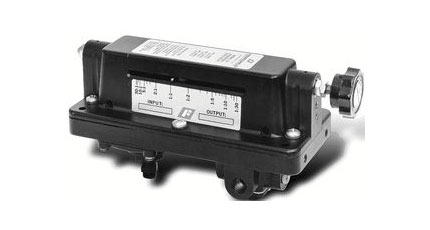Main Features

• Adjustable from 30:1 dividing ratio to 1:30 multiplying ratio.
• Venturi aspiration system.
• Optional input and output biasing.
• Floating seal ring isolates control chamber.
• Panel or Line Mounting

Benefits

• Compensates for downstream pressure losses.
• Allows versatility in applications.
• Increased stability by reducing effect of high flows.
• Easy installation and service in the field.

The Model 21 Adjustable Ratio pneumatic relay has a signal chamber lever arm, whereby the ratio of output pressure to signal pressure is infinitely adjustable. The adjustment range permits signal amplification of 1:30 or signal reduction of 30:1 by rotation of the ratio adjustment knob which moves the fulcrum. . Output pressure is a function of signal pressure times the ratio of lever arm lengths on either side of the fulcrum. Bias may be introduced by means of the set screws. The Model 21D is available with both input and output adjustable bias. Maximum input bias is 3 psig, with a maximum output bias of 9 psig. The basic mathematical expression for the bias in this relay is Po = (Ps-K1) R+K2, where Po = Output pressure, Ps = Input signal, R = Ratio of setting, K1= Input bias, (-) only, and K2 = Output bias, (+) only.

Specifications
Max Supply Pressure: 250 psig [17 BAR]
Max Output Pressure: 150 psig [10 BAR]
Flow Capacity: 40 SCFM (68 m3/HR)
Accuracy: 0.3 psi (typ)